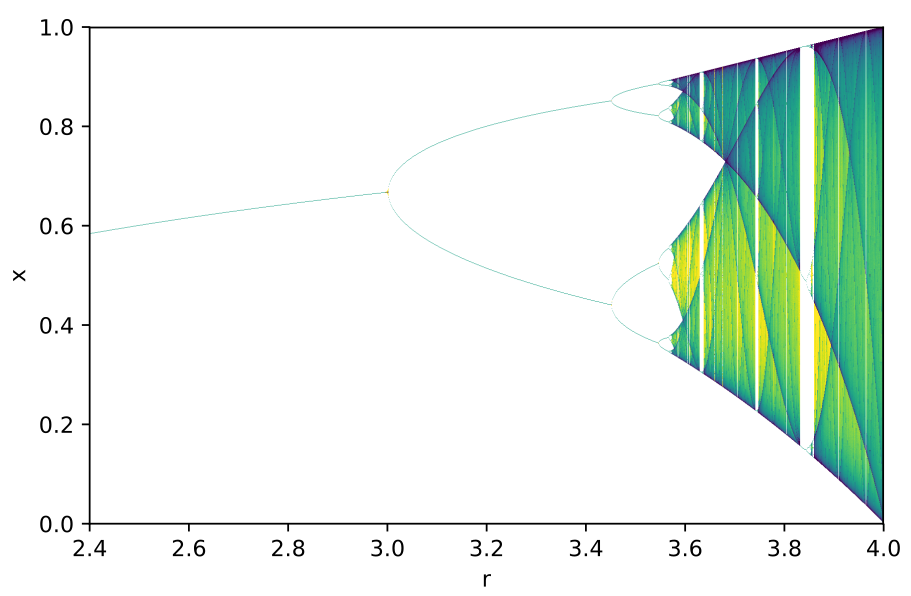# Optimization and Dynamics

The study of dynamical systems began by trying to understand the relationship between the earth and the sun. Since the birth of calculus and modern computing, the study of optimization and dynamics have exploded, bringing new mathematical perspectives to disciplines previously unknown. Both optimization and dynamics continue to be important fields of research in disciplines such as mathematics, physics, engineering, biology, chemistry, and economics.###### The bifurcation diagram of population growth.

We are using Zoom to meet and discuss virtually. We have two different Meeting IDs: one for Tuesday and one for Friday.

• Tuesday: 939-8199-5044
• Friday: 967-2582-7899

### Problem Sheets

Weekly exercise sheets are to be turned in electronically to your tutor, Dr. Lee. Unless stated otherwise, they are due Wednesdays by 23:59 (GMT+2).

### Videos

Links to videos (on YouTube) where you can find videos on topics we discuss in the course. These follow the course lecture notes.

Chapter 8

• Basics of matrix exponentials
• We look at the fundamentals of matrix exponentials. We define the expression $e^A$, or $\mathrm{exp}(A)$, and discuss how it comes up in the study of continuous time dynamical systems.
• Notes
• Properties of matrix exponentials
• We look at some basic properties of the matrix exponential, and we use them to compute more exponentials of more complicated matrices.
• Notes
• Using characteristic polynomials to compute exponentials
• This is the first video in a two part series where we use the characteristic polynomial of a matrix A to compute the exponential of A. We prove the propositions needed to apply this to matrix exponentials.
• Notes
• Examples involving characteristic polynomials
• This is the second video in the two part series on using the characteristic polynomial to compute the exponential of a matrix. Here, we apply the propositions that we proved in the first part in a few examples.
• Notes
• Analysis of linear ODE systems Part 1
• We take everything we have done in chapter 8 and use it to analyze linear systems of ODEs. We give a proof of the existence and uniqueness of solutions.
• Notes
• Analysis of linear ODE systems Part 2
• We take everything we have done in chapter 8 and use it to analyze linear systems of ODEs. We give an alternative description of a general solution to a linear ODE system, and we discuss various examples.
• Notes

Chapter 9

### Handwritten notes

PDFs of the handwritten notes made during the lecture may be uploaded.

1. Arrowsmith, D. K.; Place, C. M. An introduction to dynamical systems. Cambridge University Press, Cambridge, 1990.
2. Devaney, Robert L. An introduction to chaotic dynamical systems. Second edition. Addison-Wesley Studies in Nonlinearity. Addison-Wesley Publishing Company, Advanced Book Program, Redwood City, CA, 1989.
3. Stokey, Nancy L., Lucas, Robert E., Jr. Recursive methods in economic dynamics. With the collaboration of Edward C. Prescott. Harvard University Press, Cambridge, MA, 1989.
4. Strogatz, Steven H. Nonlinear dynamics and chaos. With applications to physics, biology, chemistry, and engineering. Second edition. Westview Press, Boulder, CO, 2015.
5. Teschl, Gerald. Ordinary differential equations and dynamical systems. Graduate Studies in Mathematics, 140. American Mathematical Society, Providence, RI, 2012.